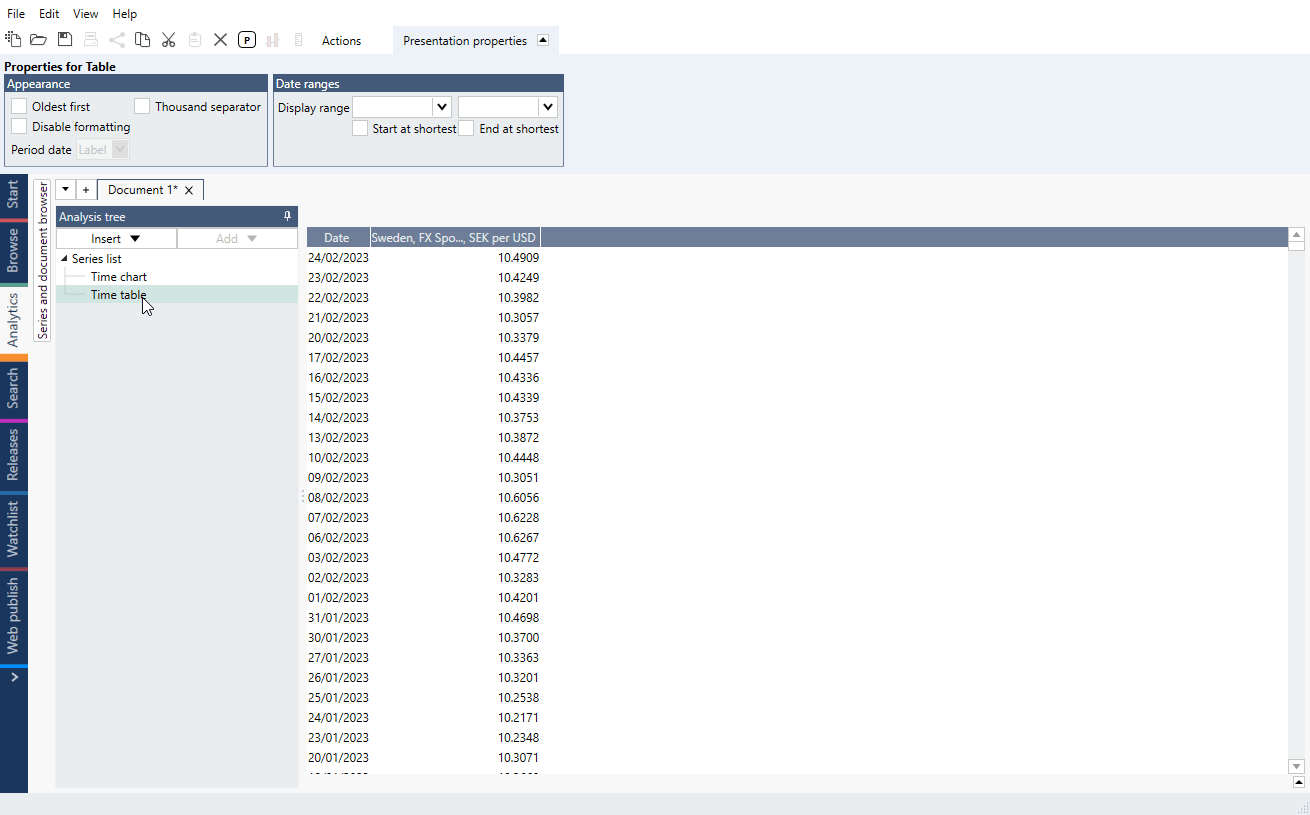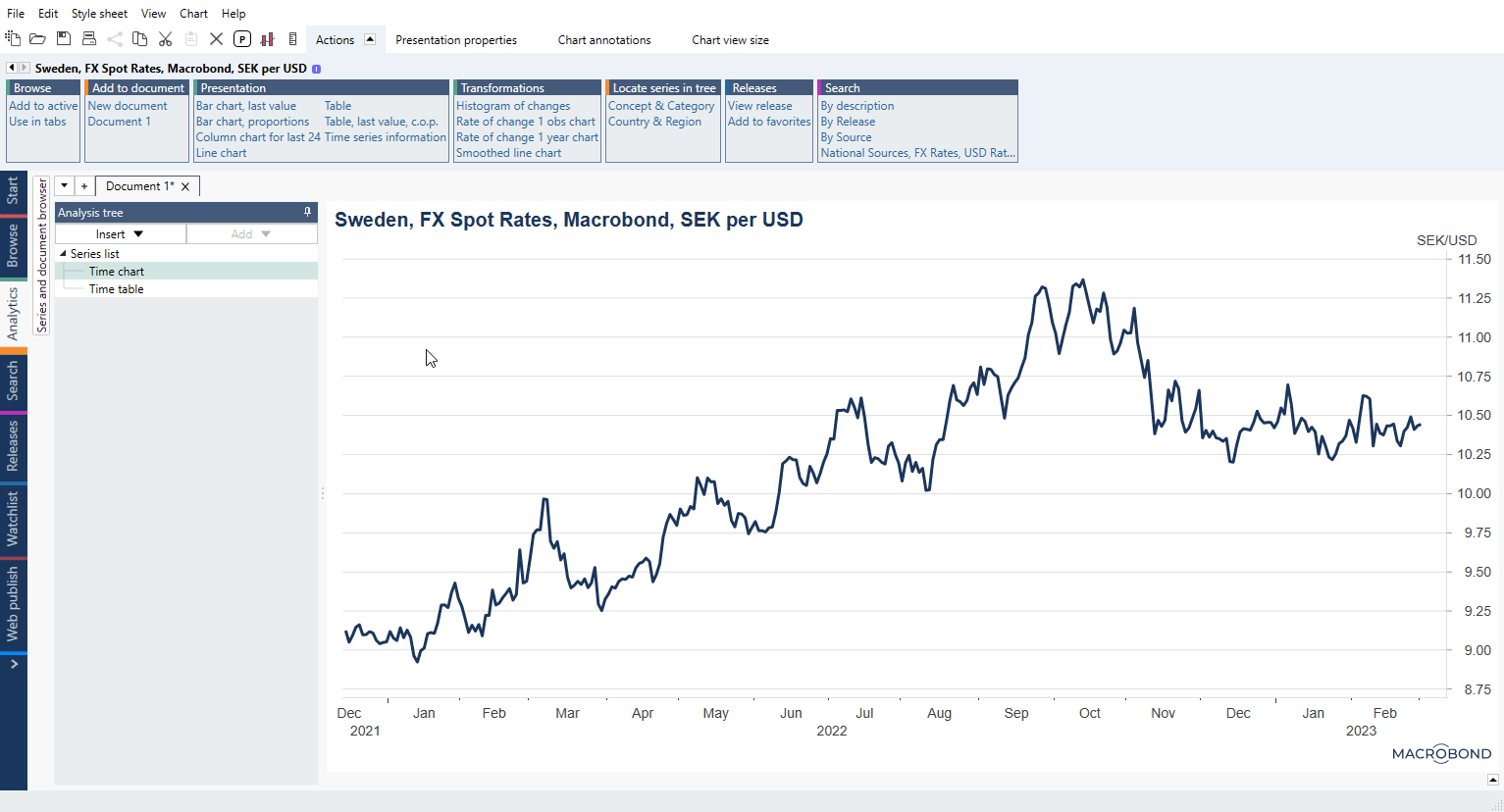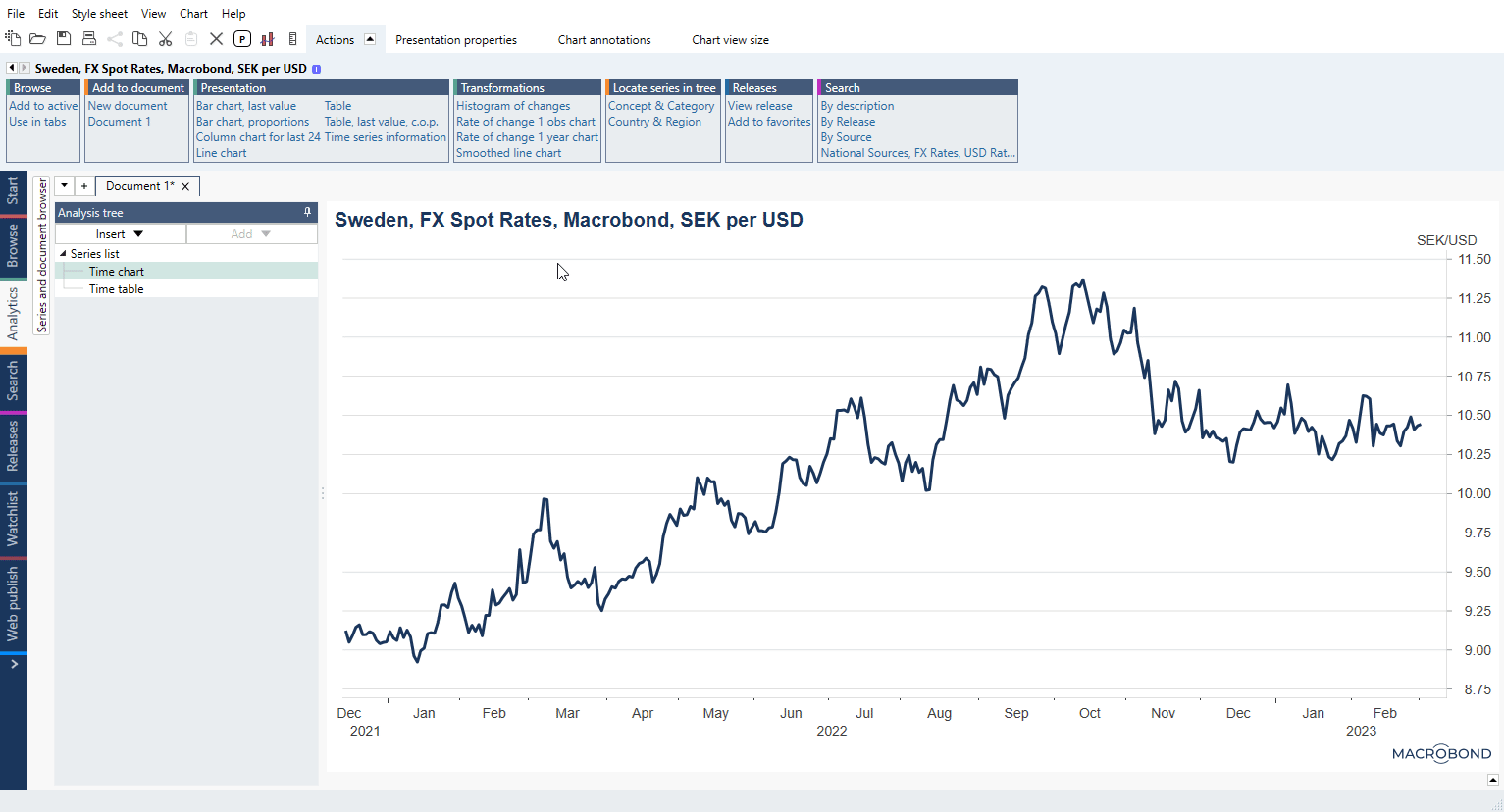# Built-in formula

## Extend()

Extend formulas - see comparison here.

### Extend with last value to the end of a period

The following formulas, which you can type into the Series list, will extend the input series to the end of the corresponding period:

ExtendToEndOfMonth(series)

ExtendToEndOfQuarter(series)

ExtendToEndOfYear(series)

If you want to mark the extended values as forecasts, use:

ExtendLastAsForecast(series)

Note series will be extended till the end of the common calendar (established at the last step before using this formula) which depends on the series included in document.

### Extend with another value to a specified point in time

Using the formula below, you can customize what value is used and until when the series is extended.

Extend(series, observation, number)

It can be translated as Extend(which series, how far, which value) and in order to work effectively you will need helper formulas like Last() or Endvalid(), for example:

Extend(uscpi, Last(EndOfYearAhead(0)), LastValid(uscpi))

will extend uscpi series to end of current year with its last value. While:

Extend(usrate0001, Endvalid(usrate0001)+Monthslength(2), 0.75)

will extend series usrate0001 and will put 0.75 value two months from its end. Observations between end of series and new point will be filled in automatically.

### Extend with YoY percentage from same series

Below formula extends the series using the % YoY change to calculate a forecast for the whole calendar range. New values will be marked automatically as forecast.

ExtendLastYoYForecast(series)

This one has an additional parameter to point to the end of extending range.

ExtendLastYoYForecast(series, observation)

Note series will be extended till the end of the common calendar (established at the last step before using this formula) which depends on the series included in document. But here you can use same method with EndValid() as in previous example:

ExtendLastYoYForecast(series, endvalid(series)+Yearslength(6))

## ExtendLinear() vs LinearExtend()

ExtendLinear vs LinearExtended - see comparison here.

Both formulas, ExtendLinear() and LinearExtend(), are used to extend the series. With similar names and purpose, they can be easily confused but they extend the series in different way.

ExtendLinear uses linear interpolation to extend the series between the latest value and a manually specified value in the future, thus extending the series with a trend.

LinearExtend uses the Least-Square method to create a fitted line, which in turn is used to extend the series to a specified point in the future.

## Join()

Join formulas - see comparison here.

### Joining two series

You can also extend one series with another. For that we have:

Join(series1, series2)

It can be translated as Join(older series, newer series). After last value of series1 Macrobond will add values from series2 from the respective points in time. This can also be modified by third parameter which will point exactly where to connect series':

Join(series1, series2, Start(series2))

Join(series1, series2, Date(yyyy, mm, dd))

### Joining two series, one with more history

We also have special join formula for appending historical values before the start of series1:

JoinMoreHistory(series1, series2)

Unlike the standard Join formula this one translates to Join(newer series, older series). It takes all observations of series1 and adds all values of series2 that are before the start of series1.

### Joining two series with different scales

For these formulas, a factor is applied to scale up the series:

JoinScaled(series1, series2)

JoinScaledAppend(series1, series2)

JoinMoreHistoryScaled(series1, series2)

JoinScaled() works like Join(). It will join series2 at the end of series1. At the date of the junction, series1 (older series) is scaled to match value of series2 (newer series). The rest of the values of series1 are then adjusted using the factor used at the date of the junction.

JoinScaledAppend() is similar to Join() and JoinScaled(). It will connect series at the end of series1, but it will scale series2 (newer series) to match series1 (older series).

JoinMoreHistoryScaled() works like JoinMoreHistory(). It will add more history to series1 (newer series) using the values of series2 (older series) that are before the start of series1. At the date of the junction, series2 (older series) is scaled to be equal to the value of series1 (newer series). The rest of the values of series2 are then adjusted using the factor used at the date of the junction.

### Joining more than two series

Join formula can connect only two series. If you need to connect more, please insert one join formula into another:

Join(series1, Join(series2, series3))

### Joining many series for different periods (but they start in same point in time)

Let's say you have series presenting values for different periods of time (i.e.; 2024, 2025, 2026 etc.) but they all start in 01/01/2023. The best way is not to use join() but moves them with formula and add them with Cross section.

Join many series with same start point

Join many series with same start point and original series

# In-app features

## Partial Periods functionality – filling in last non-complete period

If series is for example Daily and you want to convert its frequency to Annual, you will not see value for a current year because there is no 12 months of data - incomplete periods are removed.

In such situations use Conversion settings' 'Partial Periods to lower frequency method'. It will let you choose your preferred setting how to handle incomplete periods and mark the outcome as forecast value.## Forecast tab

You can add forecast to the raw time series, before any calculations are made through tab on Series list or after calculations through Forecast analysis.Note that these forecasts will be added in the original frequency of the time series, even if the document uses another frequency.

### Extend with points in time

You can add absolute values to a raw time series in the Series list, or after having applied some calculations to it. Values can be added manually or copied (vertically aligned) and pasted in.

When new values will appear forecast will be overwritten unless you change 'Value preference' in Forecast tab/analysis.### Extend relatively

Forecast can be added as absolute values for each point in time but it can be set as relative. This method will always keep your forecast ahead of existing series.# Growth/Increase by % - custom methods

## Extend based on percentage value from another series

When you have one series and you want to extend series1 based on YoY-series2 you can do it by adding values with each formula:

Extend based on YoY

Extend based on PoP

You can also extend series when future estimates are in more than one series, you just need a different approach:

Extend based on many %-series

## Growth

#### Continuous growth rate and Linear growth rate

Below example is for continuous growth rate of 5%:

AggregateProduct(1+0.05/100)*100

and this one represents Linear growth rate of 2% over time:

AggregateProduct(Pow(1+0.02, 1/round(YearLength(), 0)))*100

Continuous growth vs Linear growth - see comparison here.

#### Growth Till Date by Level

Custom formula which calculate stable growth by applied level (i.e., 1 % is expressed as 0.01) till a point in the future. That point can be also set as relative for example from the end of original series.

.GrowthTillDate(series, endDate, level) =
let
.extended=cutstart( (extend(series, endDate, LastValid(series))) , end(series))
.lastWithMultipliers = (if(counter(.extended)>end(series), 1+level, if(counter(.extended)=end(series), series, 1)))
in
join(series, flagforecast(AggregateProduct(.lastWithMultipliers)))
end

Growth Till Date by Level

#### Growth by Annual Rate

Custom formula which can calculate annual rate starting from any point in time of a series till any point in the future.

.GrowthByAnnualRate(baseSeries, annualRate, startDate, endDate) =
let
.rate = Pow(1+annualRate/100, 1/YearLength())
.base = At(baseSeries, startdate)
.period = CutStart(Extend(.base, endDate, .base), startDate)
in
.period*Pow(.rate, Counter(.period)-startDate)
end

Growth by Annual Rate

#### Growth with first point after certain value

Select a value - when series will hit this point formula will calculate growth factor. Next it will calculate future values based on this growth factor.

Growth with first point after certain value

## Increase by X% each year, month or day

We recommend using Forecast - extend relatively. With this function your forecast will always be ahead of series. It has relative weekly, monthly, quarterly and yearly setting.

### Daily

#### Series begins at Date() with value 0 and then increases 0.5/360 for every calendar day

if(Counter()>Date(2018, 04, 05), (Counter()-(Date(2018, 04, 05)))*(0.5/360), 0)

or

AggregateProduct(If (Counter()>Date(2018, 04, 05), 1+(0.5/360), 1))-1

After Date() increase by daily - see comparison here.

#### Trend lines

joinmorehistory(lag(AggregateProduct(1.5)*100, 1), 100) 50% daily growth
joinmorehistory(lag(AggregateProduct(1.2)*100, 1), 100) 20% daily growth
joinmorehistory(lag(AggregateProduct(1.1)*100, 1), 100) 10% daily growth
joinmorehistory(lag(AggregateProduct(Pow(2, 1/5))*100, 1), 100) double value every 5 days

Trend lines (x% daily growth) - see these formulas used in COVID chart.

### Increase by X% each time period

This example uses helper series created with AggregateProduct() creating new values based on increasing '1' by X%. Values are created since a particular Date() and extended till the end of original series. Then outcome is Rebased relatively by original series.

Increase by X% each time period

# Extend backward/backfill history

## Create in-house and use join

Create an Account in-house and then use join() formula to connect older values with series. This is the best option we can recommend right now.

## Join shorter series with 0

If another series has more historical values and you want to match its time frames, use formula:

join(0, series, Start(series))

Note it will add as much 0 values as there is points in time in common calendar. It won't add anything if all series start in the same moment.

## Add 100 at the start of series (for recursive formulas)

Use below formula to add 100 at the start of series:

JoinMoreHistory(100*AggregateProduct(1+fx:s1/100), 100)